# chapter1.ppt

26 de Mar de 2023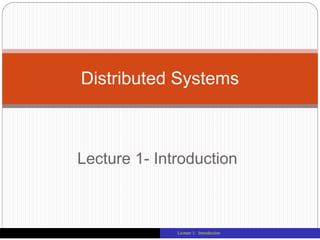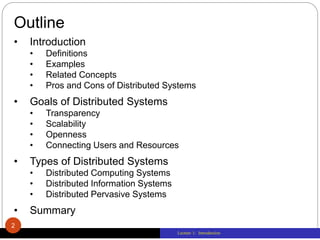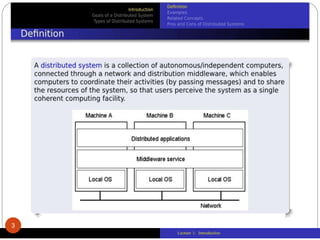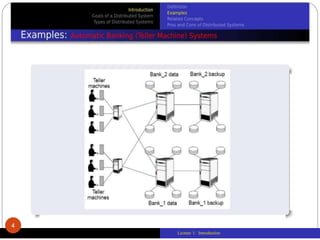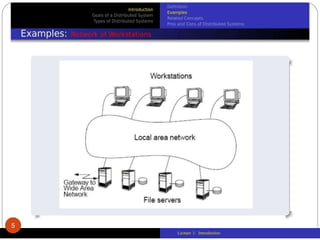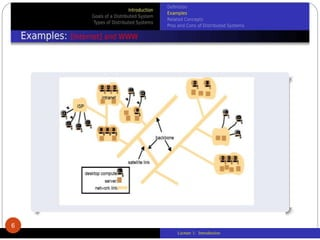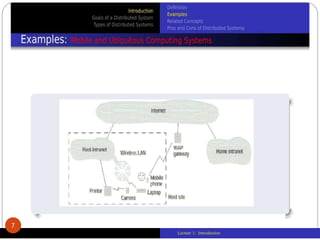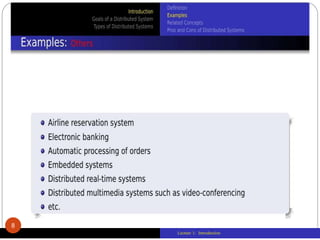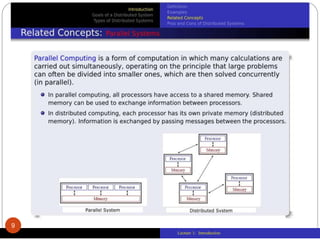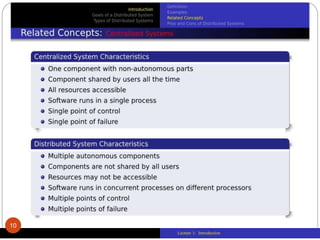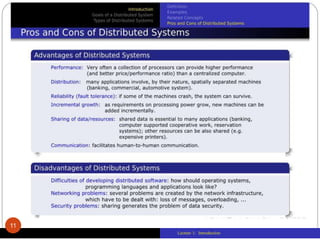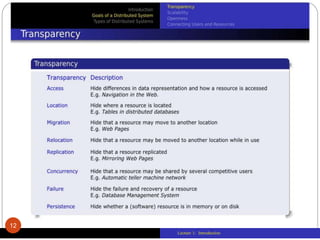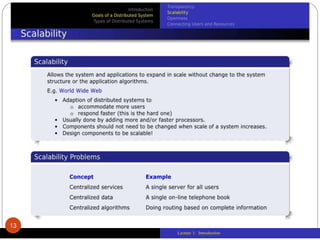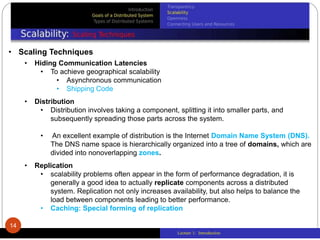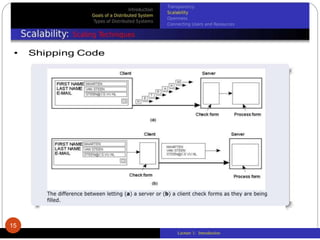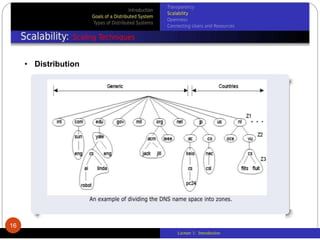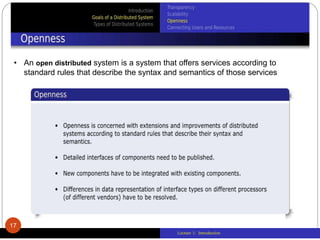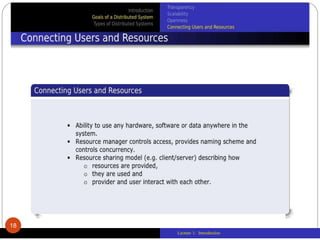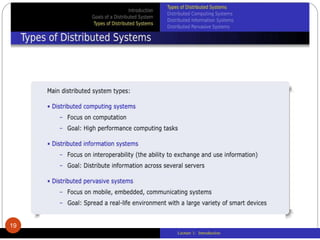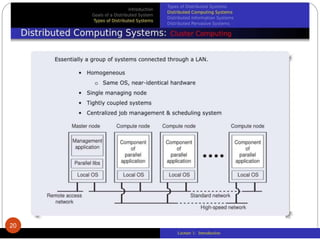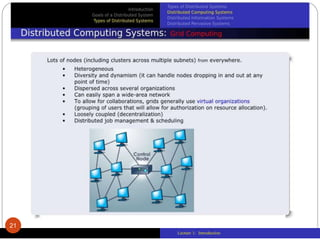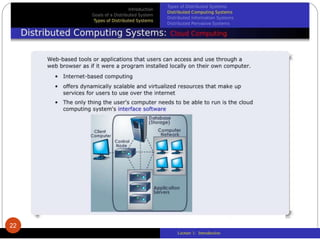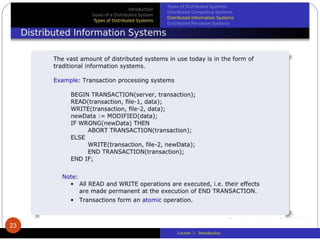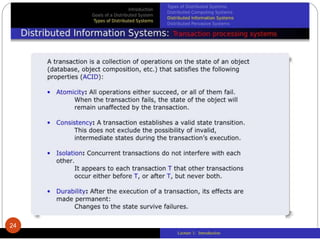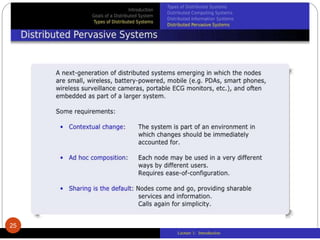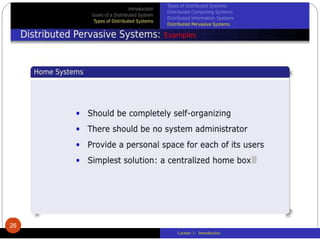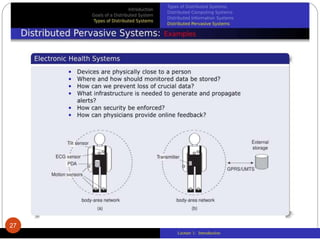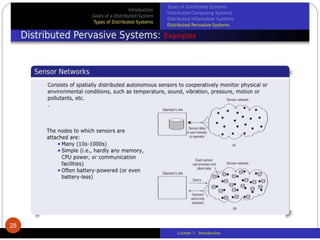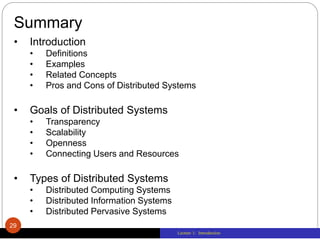1 de 29

### chapter1.ppt

1. Lecture 1: Introduction Lecture 1- Introduction Distributed Systems
2. Lecture 1: Introduction Outline • Introduction • Definitions • Examples • Related Concepts • Pros and Cons of Distributed Systems • Goals of Distributed Systems • Transparency • Scalability • Openness • Connecting Users and Resources • Types of Distributed Systems • Distributed Computing Systems • Distributed Information Systems • Distributed Pervasive Systems • Summary 2
3. Lecture 1: Introduction 3
4. Lecture 1: Introduction 4
5. Lecture 1: Introduction 5
6. Lecture 1: Introduction 6
7. Lecture 1: Introduction 7
8. Lecture 1: Introduction 8
9. Lecture 1: Introduction 9
10. Lecture 1: Introduction 10
11. Lecture 1: Introduction 11
12. Lecture 1: Introduction 12
13. Lecture 1: Introduction 13
14. Lecture 1: Introduction • Scaling Techniques • Hiding Communication Latencies • To achieve geographical scalability • Asynchronous communication • Shipping Code • Distribution • Distribution involves taking a component, splitting it into smaller parts, and subsequently spreading those parts across the system. • An excellent example of distribution is the Internet Domain Name System (DNS). The DNS name space is hierarchically organized into a tree of domains, which are divided into nonoverlapping zones. • Replication • scalability problems often appear in the form of performance degradation, it is generally a good idea to actually replicate components across a distributed system. Replication not only increases availability, but also helps to balance the load between components leading to better performance. • Caching: Special forming of replication 14
15. Lecture 1: Introduction 15
16. Lecture 1: Introduction 16 • Distribution
17. Lecture 1: Introduction 17 • An open distributed system is a system that offers services according to standard rules that describe the syntax and semantics of those services
18. Lecture 1: Introduction 18
19. Lecture 1: Introduction 19
20. Lecture 1: Introduction 20
21. Lecture 1: Introduction 21
22. Lecture 1: Introduction 22
23. Lecture 1: Introduction 23
24. Lecture 1: Introduction 24
25. Lecture 1: Introduction 25
26. Lecture 1: Introduction 26
27. Lecture 1: Introduction 27
28. Lecture 1: Introduction 28
29. Lecture 1: Introduction Summary • Introduction • Definitions • Examples • Related Concepts • Pros and Cons of Distributed Systems • Goals of Distributed Systems • Transparency • Scalability • Openness • Connecting Users and Resources • Types of Distributed Systems • Distributed Computing Systems • Distributed Information Systems • Distributed Pervasive Systems 29